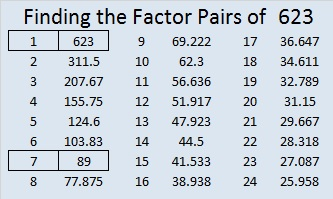# 623 and Level 2

623 is the hypotenuse of Pythagorean triple 273-560-623. What is the greatest common factor of those three numbers?

623 is not divisible by 2, 3, or 5. Is 623 divisible by 7? You can apply either of the following divisibility rules after you separate 623’s digits into 62 and 3:

1st rule: 62 – 2(3) = 62 – 6 = 56. Since 56 is divisible by 7, 623 is divisible by 7.

2nd rule: 62 + 5(3) = 62 + 15 = 77. Since 77 is divisible by 7, 623 is divisible by 7.

86 + 87 + 88 + 89 + 90 + 91 + 92 = 623  (7 consecutive numbers because 623 is divisible by 7)Print the puzzles or type the solution on this excel file: 12 Factors 2015-09-21

—————————————————————————————————

• 623 is a composite number.
• Prime factorization: 623 = 7 x 89
• The exponents in the prime factorization are 1 and 1. Adding one to each and multiplying we get (1 + 1)(1 + 1) = 2 x 2 = 4. Therefore 623 has exactly 4 factors.
• Factors of 623: 1, 7, 89, 623
• Factor pairs: 623 = 1 x 623 or 7 x 89
• 623 has no square factors that allow its square root to be simplified. √623 ≈ 24.9599679.—————————————————————————————————## 2 thoughts on “623 and Level 2”

1.nikirath

7 is the GCF, all three are divisible by 7.

2.ivasallay

You are exactly right!

This site uses Akismet to reduce spam. Learn how your comment data is processed.